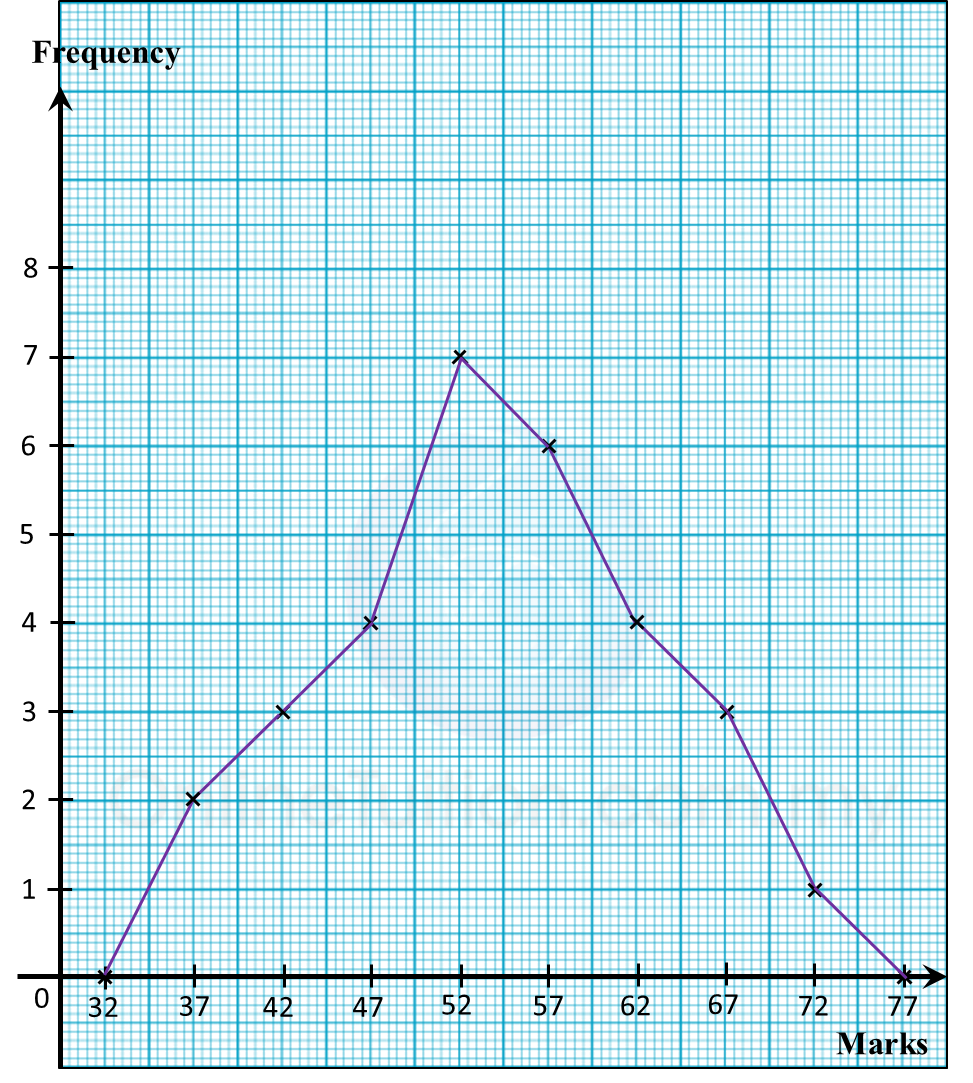# 6.8 Statistics, SPM Paper 2 (Long Questions)

Question 7:
Diagram below shows the marks obtained by a group of 30 students in a history test.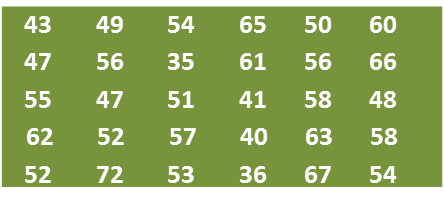(a) Using data in diagram above and a class interval of 5 marks, complete the table in the answer space.
(b) Based on the completed table in part (a)
(i) find the modal class,
(ii) calculate the mean mark obtained by the students.
(c) By using a scale of 2 cm to 10 marks on the horizontal axis and 2 cm to 1 student on the vertical axis, draw a frequency polygon for the data.

Answer: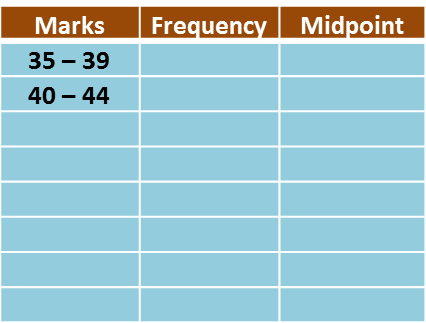Solution:
(a)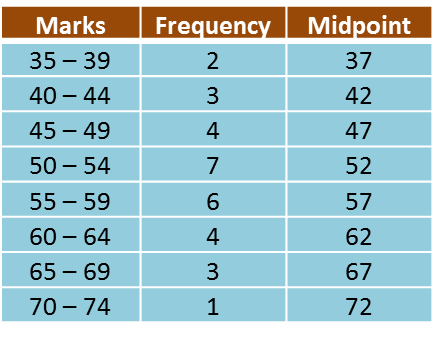(b)(i)
Modal class = 50 – 54

(b)(ii)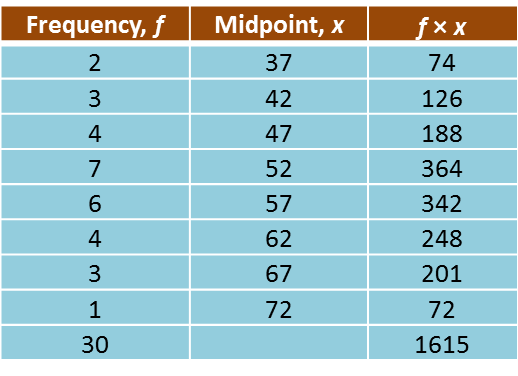(c)